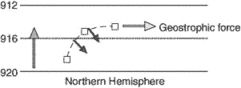# geostrophic wind speed

## geostrophic wind speedWinds exist because of horizontal and vertical pressure gradient so atmospheric motion can be deduced from isobaric surface charts etc. If the horizontal pressure gradient force is exactly balanced in magnitude by Coriolis effect accelerations of the air will be relatively small and a geostrophic wind will flow horizontally at a constant speed proportional to the isobaric spacing gradient, perpendicular to the two opposing forces and parallel to straight isobars. Thus geostrophic wind speed is proportional to the pressure gradient. The closer the isobars, the higher will be geostrophic wind speed.
The speed of a geostrophic wind calculated from the pressure gradient, air density, rotational velocity of the earth, and latitude. The calculation ignores the curvature of the wind's path. A geostrophic wind is proportional to the pressure gradient or inversely proportional to the distance between the isobars.